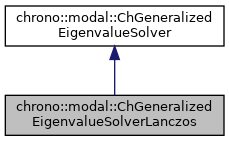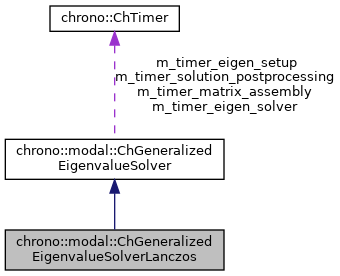chrono::modal::ChGeneralizedEigenvalueSolverLanczos Class Reference

## Description

Solves the undamped constrained eigenvalue problem with the Lanczos iterative method.

It assumes that K and M matrices are symmetric, hence a real eigenvalue problem. This is an efficient method to compute only the lower n modes, ex. when there are so many degreees of freedom that it would make a full solution impossible. It uses an iterative method and it exploits the sparsity of the matrices.

#include <ChEigenvalueSolver.h>

Inheritance diagram for chrono::modal::ChGeneralizedEigenvalueSolverLanczos:[legend]
Collaboration diagram for chrono::modal::ChGeneralizedEigenvalueSolverLanczos:[legend]

## Public Member Functions

virtual bool Solve (const ChSparseMatrix &M, const ChSparseMatrix &K, const ChSparseMatrix &Cq, ChMatrixDynamic< std::complex< double >> &V, ChVectorDynamic< std::complex< double >> &eig, ChVectorDynamic< double > &freq, ChEigenvalueSolverSettings settings=0) const override
Solve the constrained eigenvalue problem (-wsquare*M + K)*x = 0 s.t. More...

## ◆ Solve()

 bool chrono::modal::ChGeneralizedEigenvalueSolverLanczos::Solve ( const ChSparseMatrix & M, const ChSparseMatrix & K, const ChSparseMatrix & Cq, ChMatrixDynamic< std::complex< double >> & V, ChVectorDynamic< std::complex< double >> & eig, ChVectorDynamic< double > & freq, ChEigenvalueSolverSettings settings = 0 ) const
overridevirtual

Solve the constrained eigenvalue problem (-wsquare*M + K)*x = 0 s.t.

Cq*x = 0 If n_modes=0, return all eigenvalues, otherwise only the first lower n_modes.

Parameters
 M input M matrix, n_v x n_v K input K matrix, n_v x n_v Cq input Cq matrix of constraint jacobians, n_c x n_v V output matrix n x n_v with eigenvectors as columns, will be resized eig output vector with n eigenvalues, will be resized. freq output vector with n frequencies [Hz], as f=w/(2*PI), will be resized. settings optional: settings for the solver, or n. of desired lower eigenvalues. If =0, return all eigenvalues.

Implements chrono::modal::ChGeneralizedEigenvalueSolver.

The documentation for this class was generated from the following files:
• /builds/uwsbel/chrono/src/chrono_modal/ChEigenvalueSolver.h
• /builds/uwsbel/chrono/src/chrono_modal/ChEigenvalueSolver.cpp